# Shared Statistical Concepts

#### Adaptive Lasso Selection

Adaptive lasso selection is a modification of lasso selection; in adaptive lasso selection, weights are applied to each of the parameters in forming the lasso constraint (Zou 2006). More precisely, suppose that the response y has mean 0 and the regressors x are scaled to have mean 0 and common standard deviation. Furthermore, suppose that you can find a suitable estimator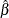of the parameters in the true model and you define a weight vector by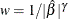, where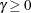. Then the adaptive lasso regression coefficients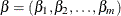are the solution to the following constrained optimization problem: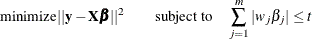PROC HPREG uses the solution to the unconstrained least squares problem as the estimator. This is appropriate unless collinearity is a concern. If the regressors are collinear or nearly collinear, then Zou (2006) suggests using a ridge regression estimate to form the adaptive weights.